# Texas Go Math Grade 2 Unit 5 Answer Key Data Analysis

Refer to our Texas Go Math Grade 2 Answer Key Pdf to score good marks in the exams. Test yourself by practicing the problems from Texas Go Math Grade 2 Unit 5 Answer Key Data Analysis.

## Texas Go Math Grade 2 Unit 5 Answer Key Data Analysis

Show What You Know

Check your understanding of important skills.

Make Tally Charts

Complete the tally chart.Explanation:
Completed the tally chartQuestion 1.
How many children chose red?
____ children
Explanation:
6 children choose red

Question 2.
Which color did the fewest children choose?
Explanation:
the fewest children choose green color

Sums to 10

Write the sum.

Question 3.
5 + 2 = ____
Explanation:
The sum of 5 and 2 is 7

Question 4.
6 + 3 = ____
Explanation:
The sum of 6 and 3 is 9

Question 5.
2 + 8 = ____
Explanation:
The sum of 2 and 8 is 10

Differences to 10

Write the difference.

Question 6.
6 – 5 = __
Explanation:
The difference of 6 and 5 is 1

Question 7.
5 – 3 = ___
Explanation:
The difference of 5 and 3 is 2

Question 8.
10 – 7 = ___
Explanation:
The difference of 10 and 7 is 3

Question 9.
9 – 2 = __
Explanation:
The difference of 9 and 2 is 7

Question 10.
8 – 4 = ___
Explanation:
The difference of 8 and 4 is 4

Question 11.
10 – 2 = ___
Explanation:
The difference of 10 and 2 is 8

Vocabulary Builder

Visualize it

Draw tally marks to show each number.Explanation:
Drawn tally marks in the table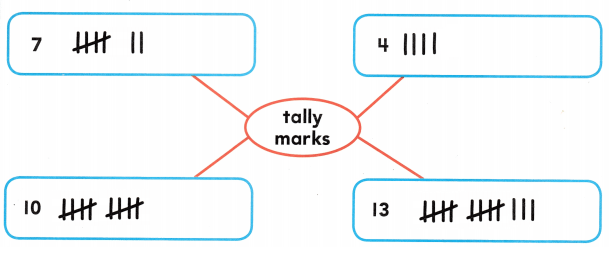Understand Vocabulary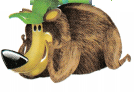Write a number to complete the sentence.

Question 1.
10 apples is more than _____ apples.
Explanation:
The word more than is used here

Question 2.
6 bananas is fewer than ___ bananas.
Explanation:
The word fewer than is used here

Question 3.
______ grapes is more than 6 grapes.
Explanation:
The word more than is used here

Question 4.
___ oranges is fewer than 5 oranges.
Explanation:
The word fewer than is used here

Field Day

written by Tim Johnson
illustrated by Lee Calderon

This Take-Home Book belongs toThe class plans a field day. They will survey 22 children to find what is liked best. They will use tally marks.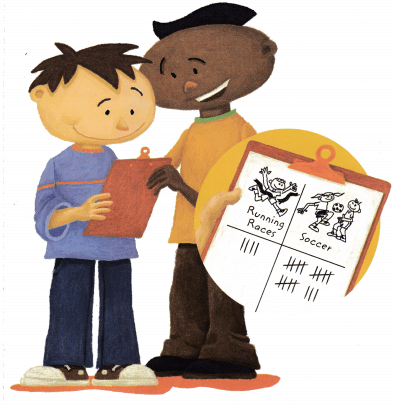Which sport was chosen most often?
soccer
Which sport was chosen least often?
Running races
Which of the two sports would you have voted for?
soccer and Running races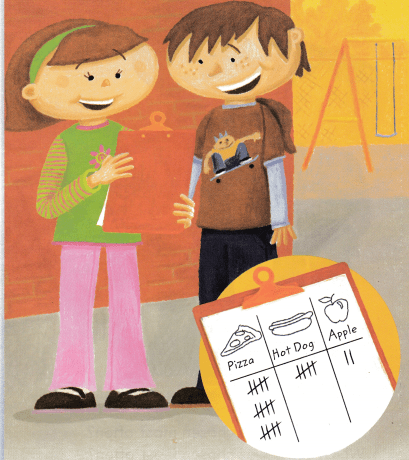What can you tell about the results of the food survey? What food would you have voted for?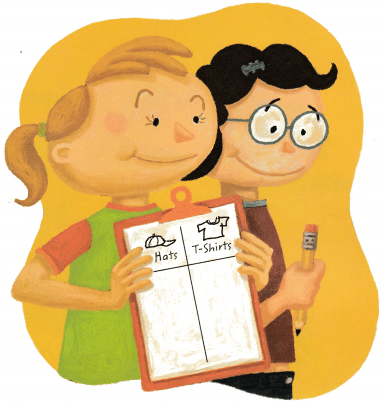This survey will show how many want hats or T-shirts. How many of the 22 children do you think ordered each item? Draw tally marks to show your idea. Remember there are 22 children ¡n all.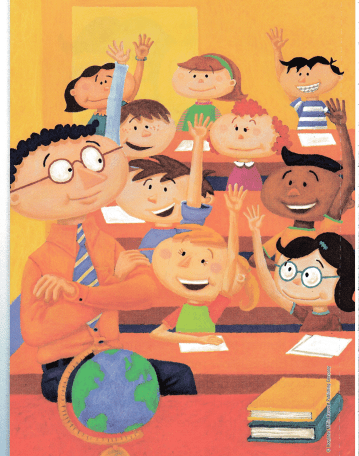The tally marks are noted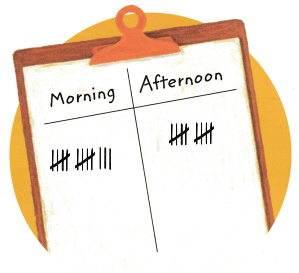Should the field day be in the morning or in the afternoon? Children raised hands to vote for morning. They kept their hands down to vote for afternoon. Tally the results.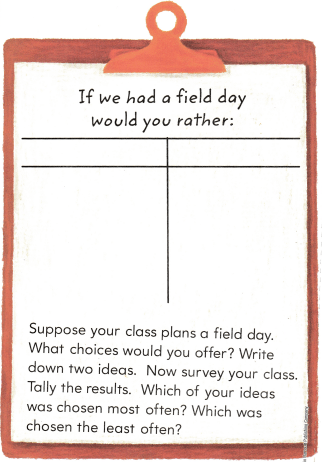Write Math Write your own story about taking a survey in your class. Draw a tally chart with tally marks to show what the results might be.Planning a Survey

Suppose your class is going to have a party. A survey is a good way to decide what to have at the party. Plan a survey about the juices or about the foods to have at the party.Question 1.
Write the three choices for your survey at the top of the tally chart.
Explanation:
Pizza, Burger, French Fries 3 options are added at the top of the tally chart.

Question 2.
What question would you use for your survey?
Answer: Which was there favorite choice
Explanation:
French Fries was the kids favorite choice for  a birthday party

Math Board Ask some classmates your survey question. Tally the results and then write to describe the results.Explanation: